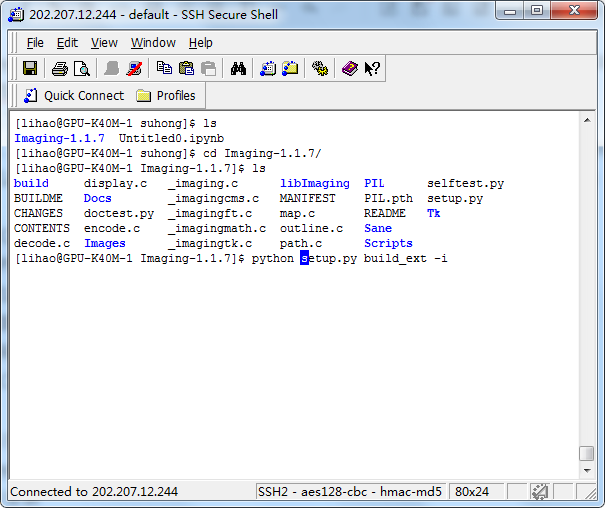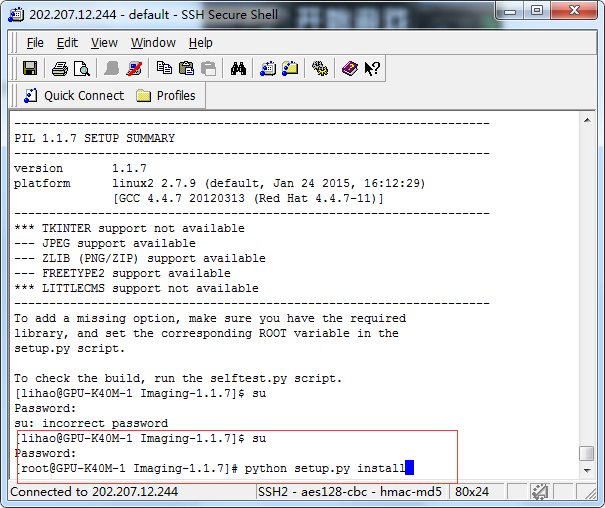• python并没有自带的图像处理库，需要独立安装，python常用的图像处理库叫做PIL(python image library). 下面介绍PIL在linux下的加载： 1.下载PIL。 2.下载并解压成功之后，到解压目录，找到 Imaging-1.1.7/setup....
python并没有自带的图像处理库，需要独立安装，python常用的图像处理库叫做PIL(python image library). 下面介绍PIL在linux下的加载：
1.下载PIL。
2.下载并解压成功之后，到解压目录，找到 Imaging-1.1.7/setup.py 这个文件，修改下面几行代码（默认TCL_ROOT的设置为NONE，这里要传到系统库的路径才行）：

TCL_ROOT = “/usr/lib64/”
JPEG_ROOT = “/usr/lib64/”
ZLIB_ROOT = “/usr/lib64/”
TIFF_ROOT = “/usr/lib64/”
FREETYPE_ROOT = “/usr/lib64/”
LCMS_ROOT = “/usr/lib64/”

3.安装前的检查
python /root/nowamagic_venv/Imaging-1.1.7/setup.py build_ext -i
4.安装
python /root/nowamagic_venv/Imaging-1.1.7/setup.py install
安装时可能没有权限，则用su命令获得管理员权限，即可完成安装展开全文pil
• python中一般使用matplotlib库进行绘图，下面我们就来看一下python中绘图时修改背景颜色的方法： 子图可以对plt.subplot的参数做修改，下面是对子图的背景颜色修改代码： import matplotlib.pyplot as plt import...
python中一般使用matplotlib库进行绘图，下面我们就来看一下python中绘图时修改背景颜色的方法：
子图可以对plt.subplot的参数做修改，下面是对子图的背景颜色修改代码：

import matplotlib.pyplot as plt

import numpy as np

# Fixing random state for reproducibility

np.random.seed(19680801)

dt = 0.01

t = np.arange(0, 30, dt)

nse1 = np.random.randn(len(t))         # white noise 1

nse2 = np.random.randn(len(t))         # white noise 2

s1 = np.sin(2 * np.pi * 10 * t) + nse1

s2 = np.sin(2 * np.pi * 10 * t) + nse2

s3 = np.sin(2 * np.pi * 10 * t) + nse1

s4 = np.sin(2 * np.pi * 10 * t) + nse2

fig= plt.figure(1) # 创建图表1

axs0=plt.subplot(221,facecolor='#FFDAB9') #在图标1中创建子图

axs0.plot(t, s1) #横轴与纵轴数据

axs0.set_xlim(0, 2) #限制x轴的取值范围

axs1=plt.subplot(222,facecolor='#7FFF00')

axs1.plot(t, s2)

axs1.set_xlim(0, 2)

axs2=plt.subplot(223,facecolor='#FF7F50')

axs2.plot(t, s3)

axs2.set_xlim(0, 2)

axs3=plt.subplot(224,facecolor='#A9A9A9')

axs3.plot(t, s4)

axs3.set_xlim(0, 2)

plt.show()

效果如下：更多Python知识请关注Python自学网。


展开全文数据可视化 机器学习 可视化 人工智能
• Mac 系统下Python绘图乱码 问题：博主在使用了Mac系统之后，发现使用Matplotlib绘图的时候，使用中文设计titile or label的时候，最终图形上并没有展现，博主查看了很多解决方案，最终找到了解决方案。 打开...
Mac 系统下Python绘图乱码

问题：博主在使用了Mac系统之后，发现使用Matplotlib绘图的时候，使用中文设计titile or label的时候，最终图形上并没有展现，博主查看了很多解决方案，最终找到了解决方案。

打开Terminal，输入以下代码：
open /Users/zbf/.matplotlib

打开的文件夹下有名称为fontlist-v330.json这样的文件，打开该文件可以查看所有Mac系统下的字体以及所对应的名称。
博主设置的中文宋体，英文Times new roman，由于本身matplotlib渲染的英文字体以及数字和Times new roman基本一致，因此可以不做设置，最终设置如下：
from matplotlib import rcParams
config = {
"font.family": 'serif',
"font.size": 12,
"font.serif": ['Songti SC'],
"mathtext.fontset": 'stix',
'axes.unicode_minus': False
}
rcParams.update(config)

折线图展开全文• 转载 http://www.th7.cn/Program/Python/201610/995260.shtml 对于label 使用 plt.legend(prop=zhfont1) 对于title等一般而言，使用 ...plt.xlabel('性别',...Matplotlib是Python的一个很好的绘图包，但是

转载 http://www.th7.cn/Program/Python/201610/995260.shtml

对于label 使用
plt.legend(prop=zhfont1)
对于title等一般而言，使用

plt.xlabel('性别',fontproperties=zhfont1)

原文

Matplotlib是Python的一个很好的绘图包，但是其本身并不支持中文（貌似其默认配置中没有中文字体），所以如果绘图中出现了中文，就会出现乱码。

在《用Python作科学计算》一书中，有一个最小二乘拟合的例子，我用Python 3.3重写的代码如下：
# 最小二乘拟合示例
import numpy as np
from scipy.optimize import leastsq
import matplotlib.pyplot as plt
import matplotlib
def func(x, p):
"""
数据拟合所用的函数: A*sin(2*pi*k*x + theta)
"""
A, k, theta = p
return A*np.sin(2*np.pi*k*x+theta)  def residuals(p, y, x):
"""
实验数据x, y和拟合函数之间的差，p为拟合需要找到的系数
"""
return y - func(x, p)
x = np.linspace(0, -2*np.pi, 100)
A, k, theta = 10, 0.34, np.pi/6 # 真实数据的函数参数
y0 = func(x, [A, k, theta]) # 真实数据
y1 = y0 + 2 * np.random.randn(len(x)) # 加入噪声之后的实验数据
p0 = [7, 0.2, 0] # 第一次猜测的函数拟合参数
# 调用leastsq进行数据拟合
# residuals为计算误差的函数
# p0为拟合参数的初始值
# args为需要拟合的实验数据
plsq = leastsq(residuals, p0, args=(y1, x))
print("真实参数:", [A, k, theta])
print("拟合参数", plsq) # 实验数据拟合后的参数
plt.plot(x, y0, label="真实数据")
plt.plot(x, y1, label="带噪声的实验数据")
plt.plot(x, func(x, plsq), label="拟合数据")
plt.legend()
plt.savefig('fit.jpg')
plt.show()

运行结果如下：
真实参数: [10, 0.34, 0.5235987755982988]
拟合参数 [ 10.02733131   0.3409059   -5.73652932]

这里图例使用的是中文，画出来的图如下：可以看出，图例中的中文并没有显示出来。

为了解决这个问题，可以使用如下方法：

因为乱码是Matplotlib缺少中文配置所导致的，所以我们只需要在程序中说明使用中文字体即可。

先选一个字体。在计算机中找到字体，选择一种中文字体，比如我这里用的是楷体右键可以查看其属性从而得知字体名称：即该字体文件为simkai.ttf

然后在程序中定义Matplotlib的字体管理，这里将其命名为zhfont1，代码如下：
zhfont1 = matplotlib.font_manager.FontProperties(fname='C:/Windows/Fonts/simkai.ttf')

接着我们只要在绘图中出现中文的地方加上字体选项即可，在最小二乘拟合的例子中，我们只需要加上语句：
plt.legend(prop=zhfont1)

完整的程序代码如下：
# 最小二乘拟合示例
import numpy as np
from scipy.optimize import leastsq
import matplotlib.pyplot as plt
import matplotlib
zhfont1 = matplotlib.font_manager.FontProperties(fname='C:/Windows/Fonts/simkai.ttf')
def func(x, p):
"""
数据拟合所用的函数: A*sin(2*pi*k*x + theta)
"""
A, k, theta = p
return A*np.sin(2*np.pi*k*x+theta)  def residuals(p, y, x):
"""
实验数据x, y和拟合函数之间的差，p为拟合需要找到的系数
"""
return y - func(x, p)
x = np.linspace(0, -2*np.pi, 100)
A, k, theta = 10, 0.34, np.pi/6 # 真实数据的函数参数
y0 = func(x, [A, k, theta]) # 真实数据
y1 = y0 + 2 * np.random.randn(len(x)) # 加入噪声之后的实验数据
p0 = [7, 0.2, 0] # 第一次猜测的函数拟合参数
# 调用leastsq进行数据拟合
# residuals为计算误差的函数
# p0为拟合参数的初始值
# args为需要拟合的实验数据
plsq = leastsq(residuals, p0, args=(y1, x))
print("真实参数:", [A, k, theta])
print("拟合参数", plsq) # 实验数据拟合后的参数
plt.plot(x, y0, label="真实数据")
plt.plot(x, y1, label="带噪声的实验数据")
plt.plot(x, func(x, plsq), label="拟合数据")
plt.legend(prop=zhfont1)
plt.savefig('fit.jpg')
plt.show()

运行后可以画出图像这样中文图例便正常显示了。

此外，在用LaTex写论文时，我们经常需要插入矢量格式的图，最好是eps或者pdf格式的图，即将存图的那行代码改为
plt.savefig('fit.pdf')

但是使用以上代码保存生成的图为pdf格式时，可能会出现错误（如果没有出现，可以无视下面的内容了），错误提示缺少第三方字体。这时我们只要把刚才的字体文件复制到Matplotlib的字体文件夹中即可，我是把Python安装到了E:/Program Files中，所以只要把simkai.ttf文件复制到如下文件夹中就ok了。
E:/Program Files/Python/Lib/site-packages/matplotlib/mpl-data/fonts/ttf

如此一来，程序便能正确输出pdf格式的图片了。

最后，再附上一个简单的例子（注：该例子改用了宋体-simsun.ttc，但图像无法存成pdf格式，但若换成simhei.ttf或者simkai.ttf均可以存成pdf格式），代码如下：
#中文标题与坐标轴示例
import matplotlib.pyplot as plt
import matplotlib
zhfont1 = matplotlib.font_manager.FontProperties(fname='C:/Windows/Fonts/simsun.ttc')
plt.xlabel('性别',fontproperties=zhfont1)
plt.ylabel('人数',fontproperties=zhfont1)
plt.title('直方图',fontproperties=zhfont1)
plt.xticks( (0,1),('男','女') ,fontproperties=zhfont1)
plt.bar(left=(0,1), height=(1,0.5), width=0.35)
plt.show()

画出的图像如下：ss

展开全文• 这篇博文主要是来介绍用python的matplotlib绘图时，如何给图形添加图例和注释。 文章目录图例指向型注释文本无指向型注释文本 图例 用于添加图例的函数是plt.legend() 指向型注释文本 用于添加指向型注释文本的...
• for i in range(5):plt.plot(x,y,col=i)如何在for循环自动更改颜色？解决方法:@tcaswell已经回答了,但是我正在键入我的答案,所以我会继续发布它…有许多不同的方法可以做到这一点.首先,matplotlib将自动循环显示...
• cdict,lut = 128) # 如果把上述语句取消注释，把LinearSegmentedColormap语句行注释掉，也可定义colormap # 但是下面语句的cmap=my_cmap应改为 cmap = "dbzcmap" ref = m.contourf(lon,lat,dbz[29,29,:,:],clevs,...
• python turtle模块简介：python2.6版本引入的一个简单的绘图工具，叫做海龟绘图(Turtle Graphics)1、.使用海龟绘图首先我们需要导入turtle：from turtle import * #将turtle的所有方法导入2、海龟绘图属性：(1)...
• 原标题：有趣的Python turtle绘图Python Turtle是Python的一个编程教育类库，越来越受到教育者的关注，近日，以"智能时代，逐梦成长”为主题的第5届全国青少年创意编程与智能设计大赛正式开始，本届大赛增加了...
• 一、既然本次讲的主角是turtle函数库，那肯定得先了解一下它是什么turtle库是Python语言一个很流行的绘制图像的函数库，想象一个小乌龟，在一个横轴为x、纵轴为y的坐标系原点，(0,0)位置开始，它根据一组函数指令...
• python turtle模块简介：python2.6版本引入的一个简单的绘图工具，叫做海龟绘图(Turtle Graphics)1.使用海龟绘图首先我们需要导入turtle，如下所示：from turtle import * #将turtle的所有方法导入2.海龟绘图...
• Python中绘图功能和MATLAB中绘图功能差不多，下面介绍一些绘图函数 由于看到了一些网友写的关于此函数的介绍，内容非常详实，所以附上链接： 一、...matplotlib
• python中matplotlib默认不支持中文，绘图时出现中文会出现各种乱七八糟的问题，这里简单整理了一点。#coding=utf-8 ''' 1.python2中要输入汉字： #coding=utf-8 2.要使用matplotlib画图： import sys reload(sys) ...
• 图形用户界面”（GUI）程序的编写——Python标准库有个turtle模块，可以生成标准的应用程序窗口进行图形绘制。turtle的绘图方式非常简单直观——想象有一只尾巴上蘸着颜料的小海龟在电脑屏幕上爬行，随着它的移动...
• Turtle库是Python语言一个很流行的绘制图像的函数库，想象一个小乌龟，在一个横轴为x、纵轴为y的坐标系原点，(0,0)位置开始，它根据一组函数指令的控制，在这个平面坐标系移动，从而在它爬行的路径上绘制了图形...编程语言
• 它可以绘制各种图形，下面就学习了下Python中的matplotlib模块，如何绘制三维图形。示例代码一：# coding=utf-8import numpy as npimport matplotlib.pyplot as pltimport mpl_toolkits.mplot3da,b = np.mgrid[-2:2....
• ## Python绘图Turtle库详解

万次阅读 多人点赞 2017-08-02 17:36:45
Turtle库是Python语言一个很流行的绘制图像的函数库，想象一个小乌龟，在一个横轴为x、纵轴为y的坐标系原点，(0,0)位置开始，它根据一组函数指令的控制，在这个平面坐标系移动，从而在它爬行的路径上绘制了图形...Turtle
• 一、既然本次讲的主角是turtle函数库，那肯定得先了解一下它是什么turtle库是Python语言一个很流行的绘制图像的函数库，想象一个小乌龟，在一个横轴为x、纵轴为y的坐标系原点，(0,0)位置开始，它根据一组函数指令...
• python matplotlib绘图 使图片能够显示中文 plt.rcParams['font.sans-serif']=['SimHei'] plt.rcParams['axes.unicode_minus'] = False 在折线图上显示数据 for a, b in zip(x1, y1): plt.text(a, b,round(b,2...可视化
• ## Python 数列绘图

千次阅读 2018-04-20 23:36:03
Python数列绘图 前言 笔者最近两天接触了Python语言...需输入的delay为输出结果的延迟时间，单位毫秒，输入的表达式可以使用random库，math库，time库所有函数，但要求符合Python语法。若要计算数列b(n)的前n项...
• Python里，海龟不仅可以画简单的黑线，还可以用它画更复杂的几何图形，用不同的颜色，甚至还可以给形状填色。一、从基本的正方形开始引入turtle模块并创建Pen对象：>>> importturtle>>> t = turtle.Pen()前面我们...
• ## python matplotlib绘图使用中文字体

万次阅读 多人点赞 2016-07-17 21:36:44
由于项目关系，需要使用到数据分析，之前有自学过python，自然想到使用python绘图。 进而接触到Matplotlib，经过几天的学习，不得不说Matplotlib是一个十分powerful的绘图工具。 下面介绍如何在绘制的matplotlib图...pyplot
• Python绘图过程总的来说就是，先把x轴和y轴的各个点对应的坐标计算出来，如果是三维的，需要计算（x,y,z）的坐标，然后描绘在坐标图上，用线连接在一起，函数图像也是一样的画法，先产生x的值，带入函数得到y的...matplotlib plot
• Python的作图软件有跟多可以做图形的开源软件包，但是在这些软件的各有各的特点，今天的mataplotlib 是一个特别强大的一个软件。它提供了一套和MATLAB相类似的绘图函数集，特别适合在用短小的一段代码来实现一......

# python中绘图python 订阅